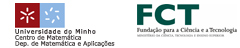EN || PT || ES# Numerical solution of a transient eddy current problem with current intensities as boundary data

The objective of this work is to analyze a time-dependent eddy current problem defined in a 3D bounded domain including conducting and dielectric materials and giving the current source in terms of current intensities. The input current intensities will be introduced in the model as non-local boundary conditions (see, for instance, ). Thus, by following , we will analyze a formulation based on the magnetic field in the conductor regions and a multivalued scalar magnetic potential in the dielectric part.

From a mathematical point of view, we will obtain a parabolic problem and prove its well posedness as well as some regularity results. We will propose a finite element combined with an implicit Euler time discretization to numerically solve the problem. Concerning the space discretization, the magnetic field is approximated by the lowest N´ed´elec edge finite elements and the magnetic potential by standard piecewise linear continuous elements. The current intensities are imposed as jumps of the multivalued magnetic potential on some prescribed cut surfaces. We will obtain convergence results for the main physical quantities, namely, the magnetic field and the current density. Finally, we will present some numerical results corresponding to the simulation of an application of electromagnetic forming.

This is a joint work with A. Bermúdez, B. López-Rodríguez, R. Rodríguez, P. Salgado.

References

 A. Bossavit, Most general non-local boundary conditions for the Maxwell equation in a bounded region, COMPEL (2000), 19, pp. 239245.

 A. Berm´udez, R. Rodr´ıguez and P. Salgado, Numerical solution of eddy current problems in bounded domains using realistic boundary conditions, Comput. Methods Appl. Mech. Engrg.© 3º Encontro Ibérico de Matemática :: 2010